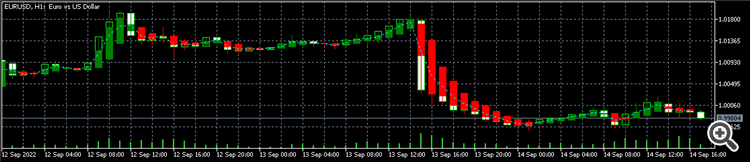# Issue accessing values of indicator buffers using CopyBuffer for indicators of type DRAW_COLOR_CANDLES

```#property tester_indicator "HAPrice.ex5"
#define LOOKBACK_LENGTH 10
#define ORDER_LOOK_BACK 2
//--- input parameters
input double   Length              = 30;
input int      MagicNumber         = 1234567;    // Expert Advisor ID
input bool     DescriptionModeFull = true;       // Detailed output mode
//--- Indicator values
double HAOpen[];
double HAHigh[];
double HALow[];
double HAClose[];
double HAAvg[];
//--- Handle of the custom indicator
int HA_handle;
//---
datetime last_bar_time = 0;
//+------------------------------------------------------------------+
//| Expert initialization function                                   |
//+------------------------------------------------------------------+
int OnInit()
{
Print(__FILE__, ": MQL Build - ", __MQLBUILD__, ", Date Time - ", __DATETIME__);
//---
ArrayResize(HAOpen, LOOKBACK_LENGTH);
ArrayResize(HAHigh, LOOKBACK_LENGTH);
ArrayResize(HALow, LOOKBACK_LENGTH);
ArrayResize(HAClose, LOOKBACK_LENGTH);
ArrayResize(HAAvg, LOOKBACK_LENGTH);
//---
ArraySetAsSeries(HAOpen, true);
ArraySetAsSeries(HAHigh, true);
ArraySetAsSeries(HALow, true);
ArraySetAsSeries(HAClose, true);
ArraySetAsSeries(HAAvg, true);
//---
const int param_count = 1;
MqlParam params[];
ArrayResize(params, param_count);
params.type = TYPE_STRING;
params.string_value = "Shared Projects\\HAPrice\\HAPrice";
//---
ResetLastError();
HA_handle = IndicatorCreate(Symbol(), Period(), IND_CUSTOM, param_count, params);
//---
if(HA_handle == INVALID_HANDLE)
{
return(INIT_FAILED);
}
else
{
return(INIT_SUCCEEDED);
}
}
//+------------------------------------------------------------------+
//| Expert deinitialization function                                 |
//+------------------------------------------------------------------+
void OnDeinit(const int reason)
{
//---
IndicatorRelease(HA_handle);
}
//+------------------------------------------------------------------+
//| Expert tick function                                             |
//+------------------------------------------------------------------+
void OnTick()
{
{
// Print("Not in trading times ...");
return;
}
//---
Print(__FILE__, ": MQL Build - ", __MQLBUILD__, ", Date Time - ", __DATETIME__);
//---
Print("---");
bool err = false;
const int start = 0;
const int num = LOOKBACK_LENGTH;
const int code0 = CopyBuffer(HA_handle, 0, start, num, HAOpen);
if(code0 < LOOKBACK_LENGTH)
{
Print("Error: HAOpen");
err = true;
}
Print("HAOpen: ", HAOpen == EMPTY_VALUE ? "Empty value" : (string) HAOpen);
const int code1 = CopyBuffer(HA_handle, 1, start, num, HAHigh);
if(code1 < LOOKBACK_LENGTH)
{
Print("Error: HAHigh");
err = true;
}
Print("HAHigh: ", HAHigh == EMPTY_VALUE ? "Empty value" : (string) HAHigh);
const int code2 = CopyBuffer(HA_handle, 2, start, num, HALow);
if(code2 < LOOKBACK_LENGTH)
{
Print("Error: HALow");
err = true;
}
Print("HALow: ", HALow == EMPTY_VALUE ? "Empty value" : (string) HALow);
const int code3 = CopyBuffer(HA_handle, 3, start, num, HAClose);
if(code3 < LOOKBACK_LENGTH)
{
Print("Error: HAClose");
err = true;
}
Print("HAClose: ", HAClose == EMPTY_VALUE ? "Empty value" : (string) HAClose);
const int code4 = CopyBuffer(HA_handle, 4, start, num, HADir);
if(code4 < LOOKBACK_LENGTH)
{
err = true;
}
const int code5 = CopyBuffer(HA_handle, 5, start, num, HAAvg);
if(code5 < LOOKBACK_LENGTH)
{
Print("Error: HAAvg");
err = true;
}
Print("HAAvg: ", HAAvg == EMPTY_VALUE ? "Empty value" : (string) HAAvg);
if(err)
{
Print("Errors");
return;
}
//---
const datetime current_bar_time = (datetime) SeriesInfoInteger(Symbol(), Period(), SERIES_LASTBAR_DATE);
if(last_bar_time == current_bar_time)
{
return;
}
last_bar_time = current_bar_time;
//---
// ...
//---
}
//+------------------------------------------------------------------+
```

I have the above code.

When I try to get the values of the indicator I get empty value. The indicator on its own works fine:```//--- indicator settings
#property indicator_chart_window
#property indicator_buffers 6
#property indicator_plots   2
#property indicator_label1  "HA open;HA high;HA low;HA close"
#property indicator_type1   DRAW_COLOR_CANDLES
#property indicator_style1  STYLE_SOLID
#property indicator_color1  clrWhite, clrRed, clrGreen
#property indicator_width1  1
#property indicator_label2  "HAAvg"
#property indicator_type2   DRAW_LINE
#property indicator_style2  STYLE_DOT
#property indicator_color2  clrAqua
#property indicator_width2  1
// ... Allocate buffers in a class onject.
//+------------------------------------------------------------------+
//| Custom indicator initialization function                         |
//+------------------------------------------------------------------+
int OnInit()
{
Print(__FILE__, ": MQL Build - ", __MQLBUILD__, ", Date Time - ", __DATETIME__);
//---
//--- Define the number of color indexes, used for a graphic plot
PlotIndexSetInteger(0, PLOT_COLOR_INDEXES, 3);
//--- Set color for each index
PlotIndexSetInteger(0, PLOT_LINE_COLOR, 0, clrWhite); // Zeroth index -> White
PlotIndexSetInteger(0, PLOT_LINE_COLOR, 1, clrRed); // First index  -> Red
PlotIndexSetInteger(0, PLOT_LINE_COLOR, 2, clrGreen); // Second index  -> Green
//---
PlotIndexSetInteger(0, PLOT_SHOW_DATA, true);
//---
return(INIT_SUCCEEDED);
}
//+------------------------------------------------------------------+
//| Custom indicator iteration function                              |
//+------------------------------------------------------------------+
int OnCalculate(const int rates_total,
const int prev_calculated,
const datetime &time[],
const double &open[],
const double &high[],
const double &low[],
const double &close[],
const long &tick_volume[],
const long &volume[],
{
//---
// ...
//---
}
//+------------------------------------------------------------------+```

Above is an outline of the indicator code.

Suminda Dharmasena:

I have deleted your other topic and copied the post below. There is no need to start a new topic with similar questions.

Suminda Dharmasena:

This follows my earlier question https://www.mql5.com/en/forum/432642

How do you use CopyBuffer with a CANDLES style indicator with a colour index when one plot has 4 buffers and a colour index? I am not getting any of the OHLC data or the colour index.

Reason: